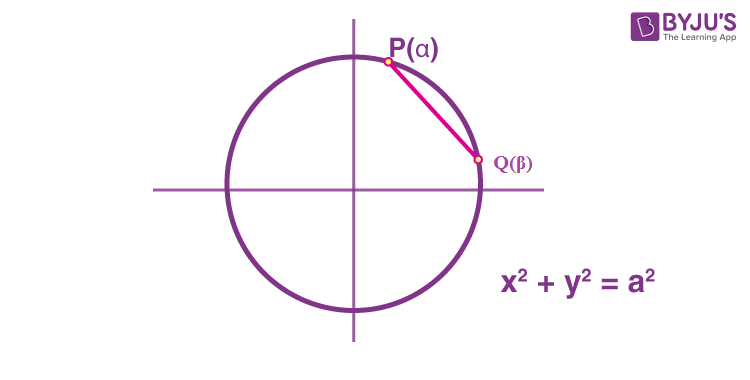# Equation of a Chord joining two points

In this article, we discuss the equation of a chord joining two points on an ellipse, hyperbola and circle. As far as any entrance exam is concerned, this is an important topic. Students can expect one question from this topic.

The line joining any two points on the circle is called the chord of the circle. The equation of the chord joining two points on an ellipse, hyperbola and circle is given below.## Formula

• Equation of the chord joining two points having eccentric angles α and β on the ellipse x2/a2 + y2/b2 = 1 is (x/a) cos (α+β)/2 + (y/b) sin (α+β)/2 = cos (α-β)/2.
• For a hyperbola, the equation of the chord joining two points P(a sec α, b tan α) and Q(a sec β, b tan β) is (x/a) cos (α-β)/2 – (y/b) sin (α+β)/2 = cos (α+β)/2.
• The equation of chord joining P(α) = (a cos α, a sin α) and Q(β) = (a cos β, a sin β) on the circle x2 + y2 = a2 is (x/a) cos (α+β)/2 + (y/a) sin (α+β)/2 = cos (α-β)/2.

### Solved Examples

Example 1:

The equation of the chord joining two points (x1,y1) and (x2,y2) on the rectangular hyperbola xy = c2 is

1) x/(x1 + x2) + y/(y1 + y2) = 1

2) x/(x1 – x2) + y/(y1 – y2) = 1

3) x/(y1 + y2) + y/(x1 + x2) = 1

4) x/(y1 – y2) + y/(x1 – x2) = 1

Solution:

Given hyperbola xy = c2

The points (x1,y1) and (x2,y2) lie on it.

So x1y1 = c2

y1 = c2/x1

(x1, y1) = (x1, c2/x1)

Similarly x2y2 = c2

y2 = c2/x2

(x2, y2) = (x2, c2/x2)

Slope of chord, m = c2(x1-x2)/x1x2(x2-x1)

= -c2/x1x2

Equation of chord is given by y – y1 = m(x-x1)

=> (y-y1)/(x-x1) = -c2/x1x2

=> x1x2y – x1x2y1 = -c2x + c2x1

=> x1x2y – c2x2 = -c2x + c2x1

=> x1x2y + c2x = c2(x2 + x1)

=> [x1x2y/c2(x2 + x1)] + c2x/c2(x2 + x1) = 1…(ii)

Also y1 + y2 = (c2/x1) + (c2/x2)

= c2(x1 + x2)/x1x2

So from (ii)

y/(y1+y2) + x/(x1 + x2) = 1

Hence, option 1 is the answer.

Example 2:

The chords joining the points θ1 and θ2 on the ellipse x2/a2 + y2/b2 = 1 intersect the major-axis in (h, 0). Prove that tan(θ1/2) tan(θ2/2) = (h-a)/(h+a)

Solution:

Equation of the chord joining θ1 and θ2 is

(x/a) cos (θ1+ θ2)/2 + (y/b) sin ( θ1+ θ2)/2 = cos ( θ1– θ2)/2

Chords pass through (h, 0).

=> (h/a) cos (θ1+ θ2)/2 + 0 = cos (θ1– θ2)/2

=> h/a = [cos (θ1– θ2)/2]/[cos (θ1+ θ2)/2]

=> (h-a)/(h+a) =[cos (θ1– θ2)/2 – cos (θ1+ θ2)/2]/[cos (θ1– θ2)/2 + cos (θ1+ θ2)/2]

= tan(θ1/2) tan (θ2/2)

Hence proved.

Related video